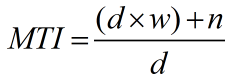Request a Tool

Mixed Number To Improper Fraction Calculator

Mixed Number To Improper Fraction Calculator is use to convert mixed number to improper fraction form.

Output

Improper Fraction
0

Formula• MTI = Mixed to Improper Fraction
• d = Values of denominator
• w = Values of whole number
• n = Values of numinator

Defination / Uses

Follow these procedures to convert a mixed number to an incorrect fraction: Add the result to the numerator after multiplying the fractional part's denominator by the entire number. As the numerator, place this result over the denominator you currently have. This approach is applicable to all mixed numbers.

Welcome to the mixed number to improper fraction calculator, a handy tool for quickly converting fractions. You've come to the correct place if you're wondering how to convert a mixed integer to an improper fraction by hand; you'll find a brief explanation below. Also, the calculator comes with a step-by-step explanation, so don't stress about your homework; we'll assist you with converting mixed integers to improper fractions! If you need to convert an improper fraction to a mixed number, use our improper fraction to mixed number calculator.